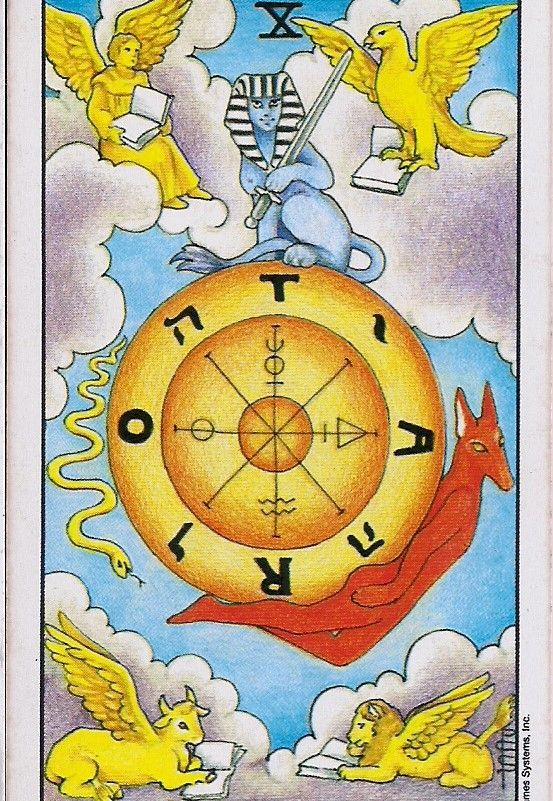# 用英日文表達「命運已經開始轉動」## wheel of fortune (wil ʌv ˈfɔrʧən) / fortune’s wheel (ˈfɔrʧənz wil)

Life (((人生))) is a wheel of fortune and it’s my turn (((輪到我))) to spin (((轉動))) it.

His (((他的))) low (((低的))) income (((收入))) life is about to (((將要))) change (((改變))) as (((當))) he turns (((轉動))) his wheel of fortune with (((藉由))) the lottery (((樂透彩))) he won (((勝出))) .

The wheel of fortune has turned in my favor (((轉而對我有利))) . Let’s (((讓我們))) see (((看))) how (((如何))) this will pan out (((進展))) .

👉

👉

👉